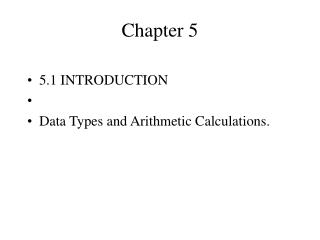Download PresentationChapter 5Chapter 5 - PowerPoint PPT Presentation

Download PresentationChapter 5
An Image/Link below is provided (as is) to download presentation

Download Policy: Content on the Website is provided to you AS IS for your information and personal use and may not be sold / licensed / shared on other websites without getting consent from its author. While downloading, if for some reason you are not able to download a presentation, the publisher may have deleted the file from their server.

- - - - - - - - - - - - - - - - - - - - - - - - - - - E N D - - - - - - - - - - - - - - - - - - - - - - - - - - -
Presentation Transcript

1. Chapter 5 • 5.1 INTRODUCTION • Data Types and Arithmetic Calculations.

2. 5.2 BASIC ARITHMETIC OPERATORS • Unary arithmetic operators require one operand. • Unary plus +, • unary minus -, • increment operator ++, • decrement operator --.

3. Binary arithmetic operators require two operands. • The computer computes an integer value for an expression in which all operands are integer.

4. 5.3 INCREMENT AND DECREMENT OPERATORS • ++ and --, • count ++; • ++ count; • count --; • -- count;

5. count=3 • print f (“%d”, count ++); • This statements print 3. • print f (“%d”, ++ count); • statements print 4.

6. 5.4 COMPOUND ASSIGNMENT OPERATORS • += -= *= /= %= • count += first; • count = count + first • They are equivalent.

7. 5.5 TYPE OF ARITHMETIC EXPRESSIONS • Example 5.8 • double first = 4.7, • int third = 27, • First + third; • Computes to 31.7, • third is converted to a floating-point value

8. Example 5.10 • Third = first + third; • A value of 31.7 is computed. • However, because the variable third is of type int, • The value of the assignment is 31.

9. Example 5.11 • X = a / 3 + b; • (a / 3) is evaluated first. • Result is 2. • The value computed for the expression is 14.5,

10. Explicit Type Conversions: The Cast Operator and Casting • The remainder operator requires integer operands. • Cast operator changes only the type of its value in temporary storage. No_of fifties = (int) amount /50; Remnant = ((int) (amount * 100) % 5000) / 100.0;

11. 5.6 MATHEMATICAL LIBRARY FUNCTIONS • Floating-point type mathematical function declarations need the directive. • Integer type mathematical functions require • The pair of parentheses, (), is known as the function call operator. #include <math.h> #include <stdlib.h>.

12. Ceil (x) • Floor (x) • Abs (x) • Fabs (x) • Sqrt (x) • Pow (x, y)

13. Sin (x) • Tan (x) • Exp (x) • Log (x) • Log 10 (x) • Figure 5.1 Some important mathematical library functions

14. 5.7 EXAMPLE PROGRAM 1:A C Program that • Solves Quadratic Equations • Requirements Specification: • Analysis: ax*x + bx+c=0 root1= root2=

15. Integer Data Types • Int : the range of –32768 through 32767 • Long integer : the range of -2,147,483,648 through 2,147,483,647. • Float : 1.175494e-38 to 3.402823e+38, • Long double: 2.22507e-308 to 1.79769e+308

16. 5.10 ARITHMETIC ERRORS AND INAACCURACIES • Important arithmetic errors • 1.Division by zero • 2.Arithmetic overflow • 3.Arithmetic underflow

17. 5.11 AUTOMATIC CONVERSION OF NUMERIC DATA TYPES • Arithmetic Conversions • The “shorter” type is converted to the “longer” type.

18. 5.12 C FEATURES FOR RANDOM NUMBER GENERATION • A random number generator is an algorithm that generates a sequence of numbers in a given range with no repeating cycles. • The first random number depends on an initial value, called the seed.

19. Rand and srand functions are in the stdlib.h header file. • Rand returns a uniform pseudorandom number in the range zero through RAND_MAX. • RAND_MAX is also found in the header file stdlib.h.

20. Every time execute program in the same environment get the same result because the function rand is a pseudorandom number generator. • Srand function is to provide a seed for the next generation of a pseudorandom number. • Time (0) is the current time.

21. #include <stdio.h> • #include <stdlib.h> • #include <time.h>

22. int main (void) { • int I = 1; • unsigned int seed; • seed = time (0); • srand (seed); • while (i <= 5) { • print f (“Throw #%d: %d\n”, I, (rand() \$ 6+1)); • I++; • } /* end while */ • return 0; • } /* end function main */